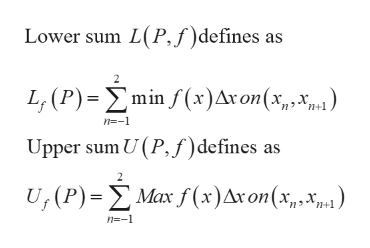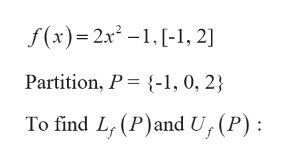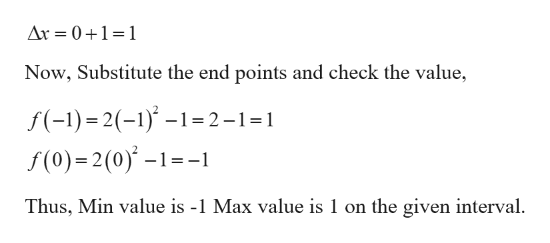# Let f(x)=2x2-1 and consider the partition {-1,0,2} of the interval [-1,2].Find Lf(P) and Uf(P).

Question
46 views

Let f(x)=2x2-1 and consider the partition {-1,0,2} of the interval [-1,2].

Find Lf(P) and Uf(P).

check_circle

Step 1

Formula used:help_outlineImage TranscriptioncloseLower sum L(P,f)defines as L; (P)=Emin f(x)Ar on(x,,x) n+1 n=-1 Upper sum U (P,f)defines as U;(P)=E Max f (x)Ax on(x,xm1) n+1 n=-1 fullscreen
Step 2

Given:help_outlineImage Transcriptionclosef(x)= 2x² -1.[-1, 2] Partition, P = {-1, 0, 2} To find L, (P)and U, (P): fullscreen
Step 3

To begin with the first partition:

...help_outlineImage TranscriptioncloseAr = 0+1=1 Now, Substitute the end points and check the value, f(-1)= 2(-1)* –1=2-1=1 f(0)=2(0) –1=-1 Thus, Min value is -1 Max value is 1 on the given interval. fullscreen

### Want to see the full answer?

See Solution

#### Want to see this answer and more?

Solutions are written by subject experts who are available 24/7. Questions are typically answered within 1 hour.*

See Solution
*Response times may vary by subject and question.
Tagged in

### Other